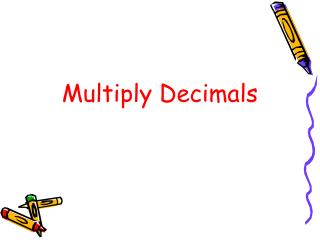DownloadDownload PresentationMultiply Decimals

# Multiply Decimals

Download Presentation## Multiply Decimals

- - - - - - - - - - - - - - - - - - - - - - - - - - - E N D - - - - - - - - - - - - - - - - - - - - - - - - - - -
##### Presentation Transcript

1. MultiplyDecimals

2. To multiply decimals: • Write the problem vertically, lining up the numbers as if they are whole numbers. Do NOT line up decimal points. • 4.8 x .31 • 4.8 • x .3 1

3. 2. Multiply as if they are whole numbers. 4.8 x .31 4.8 x .3 1 4 8 + 1 4 4 0 1 4 8 8

4. 3. Count the total number of decimal places in the factors. 4.8 x .3 1 4 8 + 1 4 4 0 1 4 4 8 Final Answer: 1.448 You have 1 decimal place in the first factor. You have 2 decimal places in the second factor. For a total of 3 decimal places.

5. Another Example: • Write the problem vertically, lining up the numbers as if they are whole numbers. Do NOT line up decimal points. • .123 x .43 • .123 • x .43

6. 2. Multiply as if they are whole numbers. .123 x .43 .123 x .43 369 + 4920 5289

7. 3. Count the total number of decimal places in the factors. .123 x .43 .123 x .43 369 + 4920 5289 You have 3 decimal places in the first factor. You have 2 decimal place in the second factor. For a total of 5 decimal places.

8. .123 x .43 369 + 4920 5289 What do I do now? I have an “empty space”? Add a zero. Final Answer: .05289 0

9. Your Turn: a) 23.1 x 4.2 =97.02 b) 1.52 x 3 =4.56